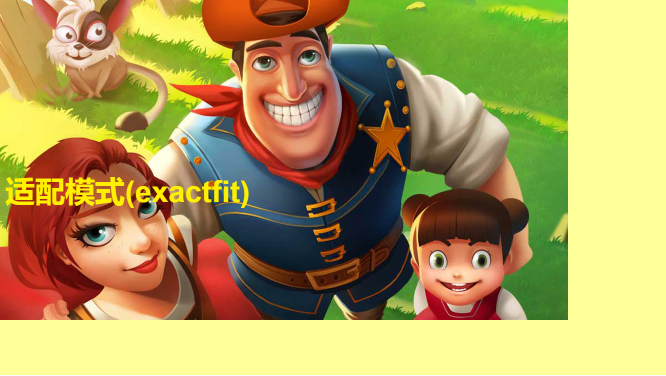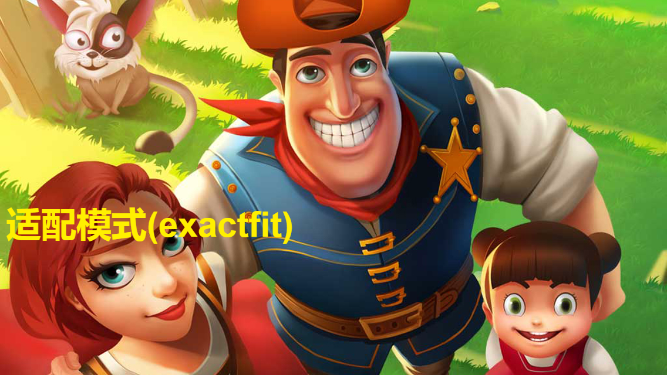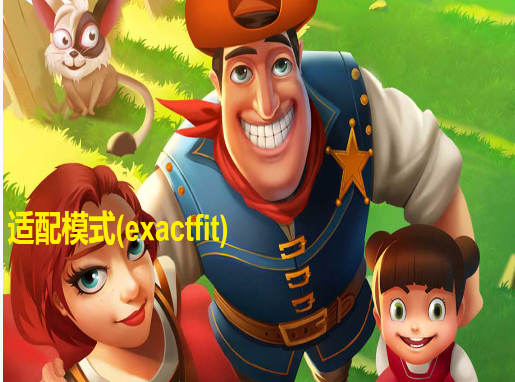# H5游戏屏幕适配的缩放模式之：拉伸填满屏幕（LayaAir引擎）

711501594

缩放模式是屏幕适配的重点内容，需要开发者通过实例体验，去深入理解。laya.display.Stage API关于缩放模式的方法scleMode共有七种参数，本篇将介绍缩放模式的拉伸填充“exactfit”。

exactfit模式是一种不考虑内容的原始比例，直接将舞台尺寸暴力拉伸，填满整个浏览器屏幕的缩放模式，下面直接上代码。

exactfit模式代码示例如下：

 123456789101112131415161718192021222324252627282930313233343536373839 `(``function``()``{``    ``var` `Stage  = Laya.Stage;``    ``var` `Text   = Laya.Text;``    ``var` `Image  = Laya.Image;` `        ``//适配模式``        ``var` `modes = ``"exactfit"``;``        ` `        ``//全局文本信息``        ``var` `txt;` `    ``(``function``()``    ``{``        ``//初始化舞台大小``        ``Laya.init(1334, 750);``        ` `        ``//设置适配模式``        ``Laya.stage.scaleMode = modes;``        ``//设置舞台背景色``        ``Laya.stage.bgColor  = ``"#ffff99"``;`  `        ``//实例一个背景``        ``var` `bg = ``new` `Image();``        ``bg.skin = ``"res/img/loadingBg.jpg"``;``        ``Laya.stage.addChild(bg);` `        ``//实例一个文本``        ``txt = ``new` `Text();``        ``txt.text = ``"适配模式("``+modes+``") "``;``        ``txt.bold = ``true``;``        ``txt.pos(10, 350);``        ``txt.fontSize = 60;``        ``txt.color   = ``"#fff000"``;``        ``Laya.stage.addChild(txt);``    ``})();` `})();`loadingBg.jpg(点击打开或另存1136, 640像素背景原图)

iPhone6横屏时运行效果如下：12 `//初始化舞台大小``Laya.init(``1136``, ``640``);`

iPhone6横屏时运行效果如下：